# Find a Missing Side Using the Cos Ratio

In this worksheet, students will use the Cos ratio to find the missing side of a triangle.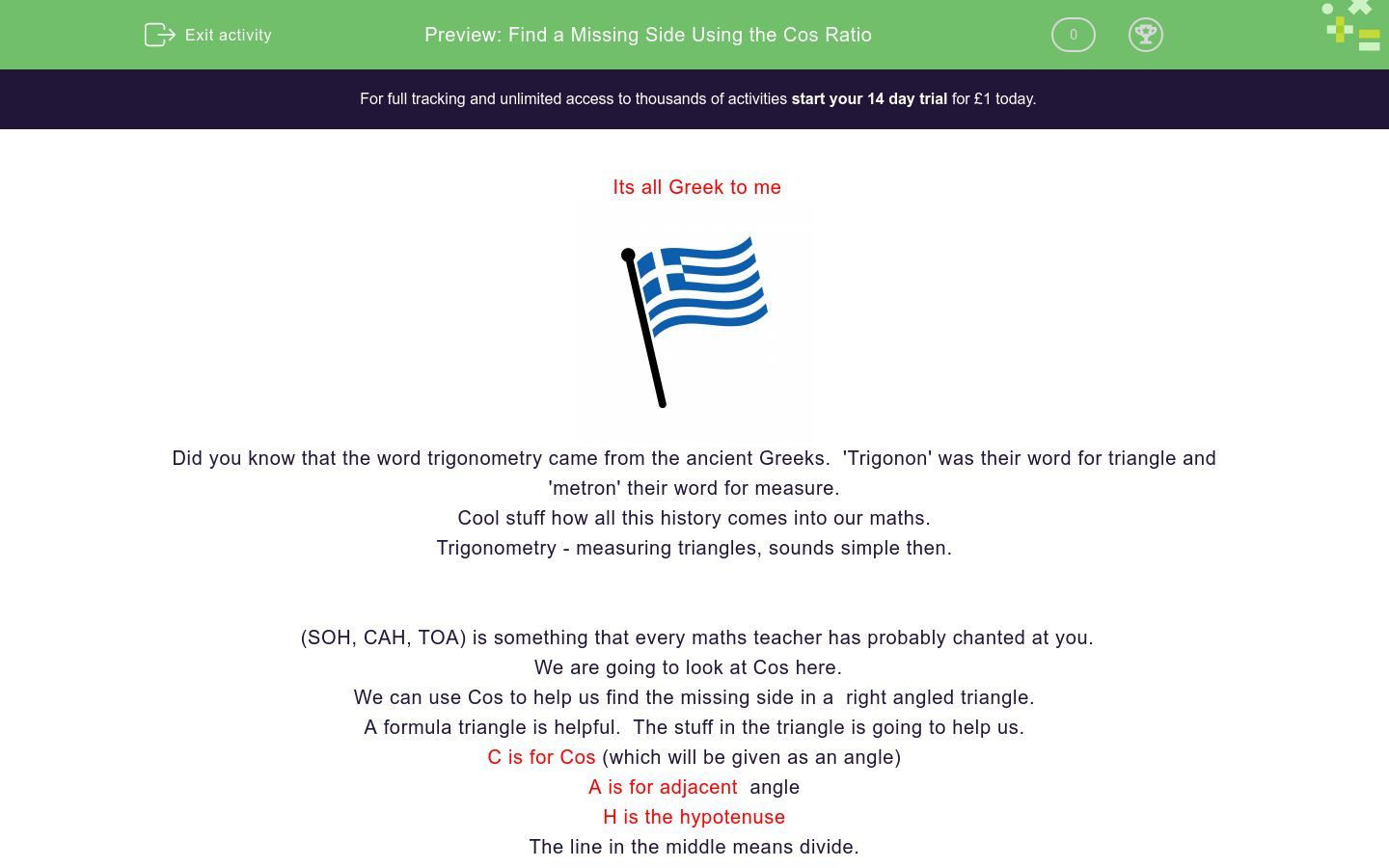Key stage:  KS 4

GCSE Subjects:   Maths

GCSE Boards:   AQA, Eduqas, OCR, Pearson Edexcel

Curriculum topic:   Geometry and Measures, Mensuration

Curriculum subtopic:   Mensuration and Calculation, Triangle Mensuration

Difficulty level:### QUESTION 1 of 10

Its all Greek to meDid you know that the word trigonometry came from the ancient Greeks.  'Trigonon' was their word for triangle and 'metron' their word for measure.

Cool stuff how all this history comes into our maths.

Trigonometry - measuring triangles, sounds simple then.

(SOH, CAH, TOA) is something that every maths teacher has probably chanted at you.

We are going to look at Cos here.

We can use Cos to help us find the missing side in a  right angled triangle.

A formula triangle is helpful.  The stuff in the triangle is going to help us.

C is for Cos (which will be given as an angle)

H is the hypotenuse

The line in the middle means divide.To use this triangle we cover up what side it is we want to find and we are left with a formula to follow.

Who would have thought formulas could be so helpful.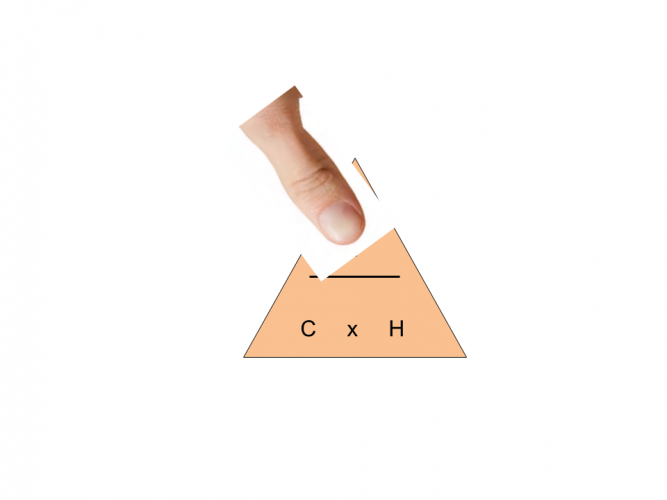If we want to find the adjacent side, cover up the A.  The formula we are left with is Cos(angle) x Hypotenuse.If we want to find the hypotenuse, cover up the H.  The formula we are left with is adjacent (A) divided by Cos(angle)Let's give it a go.

IMPORTANT NOTE:  Make sure your calculator is set to degrees.  You should see a D at the top of the screen.

Example 11. Label the triangle

2. Find the two sides you want.  You want to find x, so we want a, and the only other side you have to help is the H.

3. Look at your formula triangle, cover up the side you want to find, which is a. (see above)

4.  We are left with cos47 x 7

5. In your calculator type in  cos 47 x 7

Example 22. Find the sides you want

3.  You should have discovered you wanted H and A

4. Use your formula triangle and cover up H the side you want to find

5. The formula you are left with is adjacent ÷ cos x 10

6. 15 ÷ cos32 = 17.69 cm correct to 2 decimal places

Greek doesn't seem so bad after allFind the length marked x.

Answer has been rounded to 2 decimal places

15.56 cm

14.38 cm

14.12 cm

15.65 cmFind the value of xcos x hypotenuse adjacent divided by cos 8.12 cm 9.12 cm 8.49 cm The formula I need is The value of x iscos x hypotenuse adjacent divided by cos 8.12 cm 9.12 cm 8.49 cm The formula I need is The value of x isFind the length of x

22.25 cm

21.78 cm

27.95 cm

29.55 cm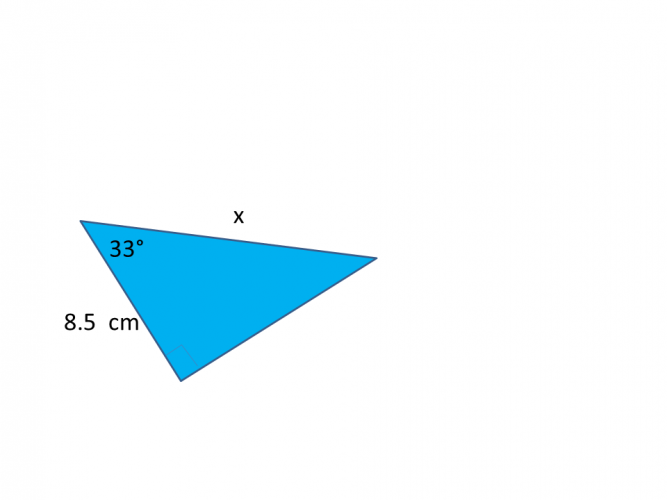cos x hypotenuse adjacent divided by cos 10.14 cm 11.24 cm 12.34 cm The formula I need is the value of x is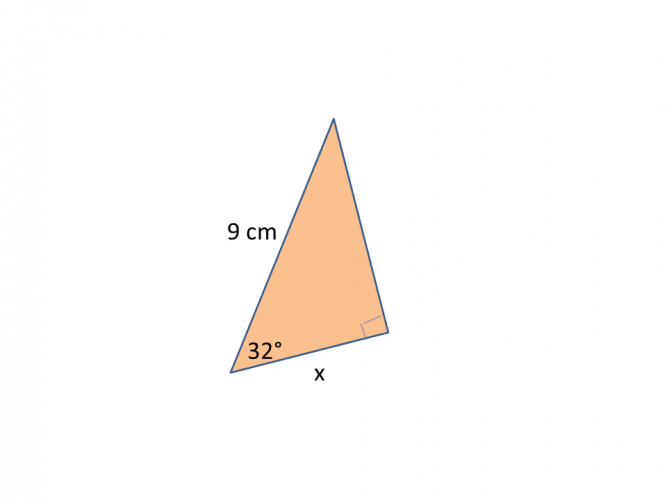cos x hypotenuse adjacent divided by cos 10.14 cm 11.24 cm 12.34 cm The formula I need is the value of x is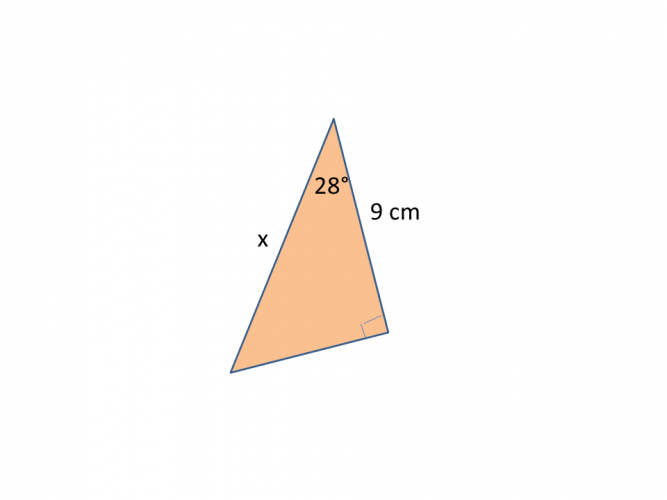Find the length of x.Find the value of x.

Answer has been rounded to 2 decimal places (2 d.p)

29.47 cm

28..82 cm

33.32 cm

35.22 cm

• Question 1Find the length marked x.

Answer has been rounded to 2 decimal places

15.56 cm
EDDIE SAYS
The tricky part of the question is labelling your triangle correctly. Why do you have to label the triangle? Just be cos.... You should have discovered that you needed the adjacent divided by cos 45. 11 ÷ cos 45 = 15.56 cm (to 2 d.p)
• Question 2Find the value of x

3.1
EDDIE SAYS
How are you going with the labelling of the triangle? It can get tricky I know. You should have discovered that you needed the Cos x the hypotenuse. Cos 62 x 6.5 = 3.05 (3.1 to 1 d.p)
• Question 3cos x hypotenuse adjacent divided by cos 8.12 cm 9.12 cm 8.49 cm The formula I need is The value of x is
EDDIE SAYS
Why didn't the sin and tan get on? Just cos... You should have discovered you needed cos and the hypotenuse. cos45 x 12 = 8.49 cm to 2 decimal places
• Question 4EDDIE SAYS
How are you getting on using the formula triangle? For this one you needed to find the adjacent so the formula was cos33 x 21 = 17.61 cm
• Question 5Find the length of x

21.78 cm
EDDIE SAYS
Are you getting used to this now? This was a multiplication as we wanted to find the opposite. adjacent ÷ cos50 14 ÷ cos50 = 21.78 cm to (2 d.p). When finding a side we always need the angle, it is just a case of deciding if we multiply or divide with it.
• Question 626.43
EDDIE SAYS
Here we need adjacent ÷ cos. 12 ÷ cos 63 7 = 26.43 cm (to 2 d.p)
• Question 7cos x hypotenuse adjacent divided by cos 10.14 cm 11.24 cm 12.34 cm The formula I need is the value of x is
EDDIE SAYS
To be honest once you can label your triangle correctly you can leave the rest to your scientific calculator. What a great friend it is at a time like this. 8.5 ÷ cos33 = 10.14 cm
• Question 8EDDIE SAYS
Calculators rock. The only thing you have to be careful of, is rounding to the correct number of decimal places. cos 32 x 9 = 7.63 cm (to 2 d.p)
• Question 9Find the length of x.

10.19
EDDIE SAYS
Nearly there. I hope you haven't found this to tri-ing!! adjacent ÷ cos28 9 ÷ cos28 = 10.19 (to 2 d.p)
• Question 10Find the value of x.

Answer has been rounded to 2 decimal places (2 d.p)

35.22 cm
EDDIE SAYS
And finally, just cos...... One final check though cos33 x 42 = 35.22 cm (to 2 d.p)
---- OR ----

Sign up for a £1 trial so you can track and measure your child's progress on this activity.

### What is EdPlace?

We're your National Curriculum aligned online education content provider helping each child succeed in English, maths and science from year 1 to GCSE. With an EdPlace account you’ll be able to track and measure progress, helping each child achieve their best. We build confidence and attainment by personalising each child’s learning at a level that suits them.

Get started